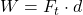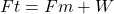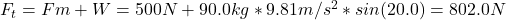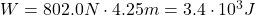## Calculate the work done (in J) by a 90.0 kg man who pushes a crate 4.25 m up along a ramp that makes an angle of 20.0° with the horizontal (

Question

Calculate the work done (in J) by a 90.0 kg man who pushes a crate 4.25 m up along a ramp that makes an angle of 20.0° with the horizontal (see below). He exerts a force of 535 N on the crate parallel to the ramp and moves at a constant speed. Be certain to include the work he does on the crate and on his body to get up the ramp.

in progress 0
4 months 2021-09-04T11:58:44+00:00 1 Answers 4 views 0

W = 3.4×0³ J.

Explanation:

The work done by the man is given by the following equation:(1)

where W: is the work, Ft is the total force and d: is the displacement = 4.25 m.

We need to find first the total force Ft, which is:where Fm: is the force exerted by the man = 535 N, W: is the weight = m*g*sin(θ), m: is the mass of the man, g: is the gravitational acceleration = 9.81 m/s², and θ: is the angle = 20.0°.Hence, the work is:Therefore, the work done by the man is 3.4×10³ J.

I hope it helps you!